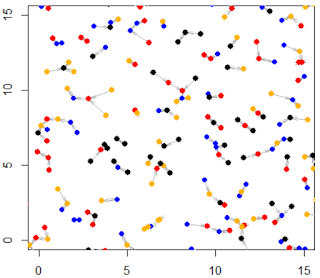Sunday, March 1, 2020

State-of-the-Art Statistical Science to Tackle Famous Number Theory Conjectures

The methodology described here has broad applications, leading to new statistical tests, new type of ANOVA (analysis of variance), improved design of experiments, interesting fractional factorial designs, a better understanding of irrational numbers leading to cryptography, gaming and Fintech applications, and high quality random numbers generators (and when you really need them). It also features exact arithmetic / high performance computing and distributed algorithms to compute millions of binary digits for an infinite family of real numbers, including detection of auto- and cross-correlations (or lack of) in the digit distributions.
The data processed in my experiment, consisting of raw irrational numbers (described by a new class of elementary recurrences) led to the discovery of unexpected apparent patterns in their digit distribution: in particular, the fact that a few of these numbers, contrarily to popular belief, do not have 50% of their binary digits equal to 1. It turned out that perfectly random digits simulated in large numbers, with a good enough pseudo-random generator, also exhibit the same strange behavior, pointing to the fact that pure randomness may not be as random as we imagine it is. Ironically, failure to exhibit these patterns would be an indicator that there really is a departure from pure randomness in the digits in question.
In addition to new statistical / mathematical methods and discoveries and interesting applications, you will learn in my article how to avoid this type of statistical traps that lead to erroneous conclusions, when performing a large number of statistical tests, and how to not be misled by false appearances. I call them statistical hallucinations and false outliers.
This article has two main sections: section 1, with deep research in number theory, and section 2, with deep research in statistics, with applications. You may skip one of the two sections depending on your interests and how much time you have. Both sections, despite state-of-the-art in their respective fields, are written in simple English. It is my wish that with this article, I can get data scientists to be interested in math, and the other way around: the topics in both cases have been chosen to be exciting and modern. I also hope that this article will give you new powerful tools to add to your arsenal of tricks and techniques. Both topics are related, the statistical analysis being based on the numbers discussed in the math section.
One of the interesting new topics discussed here for the first time is the cross-correlation between the digits of two irrational numbers. These digit sequences are treated as multivariate time series. I believe this is the first time ever that this subject is not only investigated in detail, but  in addition comes with a deep, spectacular probabilistic number theory result about the distributions in question, with important implications in security and cryptography systems. Another related topic discussed here is a generalized version of the Collatz conjecture, with some insights on how to potentially solve it.
Content
1. On the Digits Distribution of Quadractic Irrational Numbers
• Properties of the recursion
• Reverse recursion
• Properties of the reverse recursion
• Connection to Collatz conjecture
• Source code
• New deep probabilistic number theory results
• Spectacular new result about cross-correlations
• Applications
2. New Statistical Techniques Used in Our Analysis
• Data, features, and preliminary analysis
• Doing it the right way
• Are the patterns found a statistical illusion, or caused by errors, or real?
• Pattern #1: Non-Gaussian behavior
• Pattern #2: Illusionary outliers
• Pattern #3: Weird distribution for block counts
• Related articles and books
Appendix